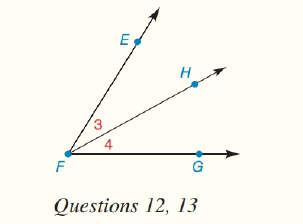Chapter 1.CT, Problem 12CT### Elementary Geometry for College St...

6th Edition
Daniel C. Alexander + 1 other
ISBN: 9781285195698

#### Solutions

Chapter
Section### Elementary Geometry for College St...

6th Edition
Daniel C. Alexander + 1 other
ISBN: 9781285195698
Textbook Problem
1 views

# In the figure, m ∠ E F G = 68 ° and m ∠ 3 = 33 ° . Find m ∠ 4 .________To determine

To find:

The value of m4.

Explanation

Given:

The given figure is,

And, mEFG=68° and m3=33°

Approach:

According to Angle-Addition postulate, if point B lies in the interior of AOC then, mAOB+mBOC=mAOC.

Calculation:

mEFG=m3+m4

### Still sussing out bartleby?

Check out a sample textbook solution.

See a sample solution

#### The Solution to Your Study Problems

Bartleby provides explanations to thousands of textbook problems written by our experts, many with advanced degrees!

Get Started

#### Find more solutions based on key concepts